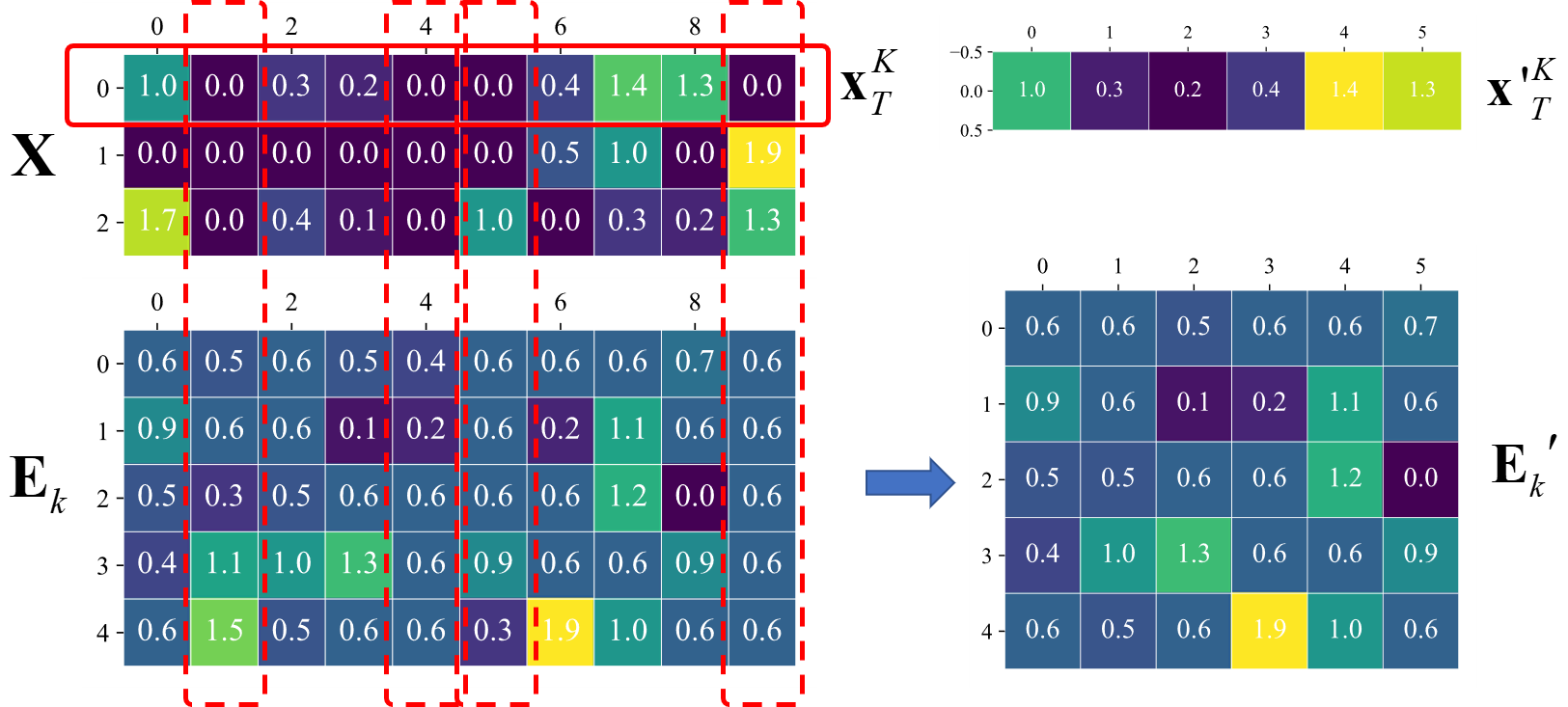# 1、字典学习思想

1. 我们通常会要求的我们的字典尽可能全面，也就是说总结出的字典不能漏下关键的知识点。
2. 查字典的时候，我们想要我们查字典的过程尽可能简洁，迅速，准确。即，查字典要快、准、狠。
3. 查到的结果，要尽可能地还原出原来知识。当然，如果要完全还原出来，那么这个字典和查字典的方法会变得非常复杂，所以我们只需要尽可能地还原出原知识点即可。

# 2、字典学习数学模型

## 2.1 数学描述

• “以前的知识”，更专业一点，我们称之为原始样本，用矩阵$\mathbf{Y}$表示；
• “字典”，我们称之为字典矩阵，用$\mathbf{D}$表示，“字典”中的词条，我们称之为原子（atom），用列向量$\mathbf{d}_k$表示；
• “查字典的方法”，我们称为稀疏矩阵，用$\mathbf{X}$
• “查字典的过程”，我们可以用矩阵的乘法来表示，即$\mathbf{DX}$

$\min_{\mathbf{D,\ X}}{\|\mathbf{Y}-\mathbf{DX}\|^2_F},\quad \text{s.t.}\ \forall i,\ \|\mathbf{x}_i\|_0 \le T_0 \tag{2-1}$

$\min_{\mathbf{D,\ X}}\sum_i\|\mathbf{x}_i\|_0, \quad \text{s.t.}\ \min_{\mathbf{D,\ X}}{\|\mathbf{Y}-\mathbf{DX}\|^2_F} \le \epsilon, \tag{2-2}$

## 2.2 求解问题

$\min_{\mathbf{D,\ X}}{\|\mathbf{Y}-\mathbf{DX}\|^2_F}+\lambda\|\mathbf{x}_i\|_1 \tag{2-3}$

\begin{aligned} {\|\mathbf{Y}-\mathbf{DX}\|^2_F} =&\left\|\mathbf{Y}-\sum^K_{j=1}\mathbf{d}_j\mathbf{x}^j_T\right\|^2_F \\ =&\left\|\left(\mathbf{Y}-\sum_{j\ne k}\mathbf{d}_j\mathbf{x}^j_T\right)-\mathbf{d}_k\mathbf{x}^k_T\right\|^2_F\\ =&\left\|\mathbf{E}_k - \mathbf{d}_k\mathbf{x}_T^k \right\|^2_F \end{aligned} \tag{2-4}

$\min_{\mathbf{d}_k,\ \mathbf{x}^k_T}\left\|\mathbf{E}_k - \mathbf{d}_k\mathbf{x}_T^k \right\|^2_F$$\min_{\mathbf{d}_k,\ \mathbf{x}^k_T}\left\|\mathbf{E}_k^{'} - \mathbf{d}_k\mathbf{x{'}}_T^{k} \right\|^2_F \tag{2-5}$

$\mathbf{E}_k^{'}=U\Sigma V^T \tag{2-6}$

## 2.3 字典学习算法实现

2.2小节，利用稀疏算法求解得到稀疏矩阵$\mathbf{X}$后，逐列更新字典，有如下算法1.1。

1. 初始化： 从原始样本$Y \in \mathbf{R}^{m \times n}$随机取$K$个列向量或者取它的左奇异矩阵的前$K$个列向量$\{\mathbf{d}_1,\mathbf{d}_2,\cdots,\mathbf{d}_K\}$作为初始字典的原子，得到字典$\mathbf{D}^{(0)} \in \mathbf{R}^{m \times K}$。令$j=0$，重复下面步骤2-3，直到达到指定的迭代步数，或收敛到指定的误差：

2. 稀疏编码： 利用字典上一步得到的字典$\mathbf{D}^{(j)}$，稀疏编码，得到$\mathbf{X}^{(j)}\in\mathbf{R}^{K \times n}$

3. 字典更新： 逐列更新字典$\mathbf{D}^{(j)}$，字典的列$\mathbf{d}_k \in \{\mathbf{d}_1,\mathbf{d}_2,\cdots,\mathbf{d}_K\}$

• 当更新$\mathbf{d}_k$时，计算误差矩阵$\mathbf{E}_k$

$\mathbf{E}_k=\mathbf{Y}-\sum_{j\ne k}\mathbf{d}_j\mathbf{x}^j_T.$

• 取出稀疏矩阵第$k$个行向量$\mathbf{x}^k_T$不为0的索引的集合$\omega_k = \{i|1\le i\le n,\ \mathbf{x}_T^k(i) \ne 0\}$$\mathbf{x'}_T^{k} = \{\mathbf{x}_T^k(i)|1\le i\le n,\ \mathbf{x}_T^k(i) \ne 0\}$

• $\mathbf{E}_k$取出对应$\omega_k$不为0的列，得到$\mathbf{E}_k^{'}$.

• $\mathbf{E}_k^{'}$作奇异值分解$\mathbf{E}_k=U\Sigma V^T$，取$U$的第1列更新字典的第$k$列，即$\mathbf{d}_k=U(\cdot,1)$；令$\mathbf{x'}^k_T=\Sigma(1,1)V(\cdot,1)^T$，得到$\mathbf{x{'}}^k_T$后，将其对应地更新到原$\mathbf{x}_T^k$

• $j = j + 1$

# 3、字典学习Python实现

## 载入数据

import numpy as np
import pandas as pd

train_data = train_data_mat["Data"]
train_label = train_data_mat["Label"]

print(train_data.shape, train_label.shape)


## 初始化字典

u, s, v = np.linalg.svd(train_data)
n_comp = 50
dict_data = u[:, :n_comp]


## 字典更新

def dict_update(y, d, x, n_components):
"""
使用KSVD更新字典的过程
"""
for i in range(n_components):
index = np.nonzero(x[i, :])
if len(index) == 0:
continue
# 更新第i列
d[:, i] = 0
# 计算误差矩阵
r = (y - np.dot(d, x))[:, index]
# 利用svd的方法，来求解更新字典和稀疏系数矩阵
u, s, v = np.linalg.svd(r, full_matrices=False)
# 使用左奇异矩阵的第0列更新字典
d[:, i] = u[:, 0]
# 使用第0个奇异值和右奇异矩阵的第0行的乘积更新稀疏系数矩阵
for j,k in enumerate(index):
x[i, k] = s * v[0, j]
return d, x


## 迭代更新求解

from sklearn import linear_model

max_iter = 10
dictionary = dict_data

y = train_data
tolerance = 1e-6

for i in range(max_iter):
# 稀疏编码
x = linear_model.orthogonal_mp(dictionary, y)
e = np.linalg.norm(y - np.dot(dictionary, x))
if e < tolerance:
break
dict_update(y, dictionary, x, n_comp)

sparsecode = linear_model.orthogonal_mp(dictionary, y)

train_restruct = dictionary.dot(sparsecode)

posted @ 2018-12-09 13:57  EndlessCoding  阅读(51660)  评论(44编辑  收藏  举报